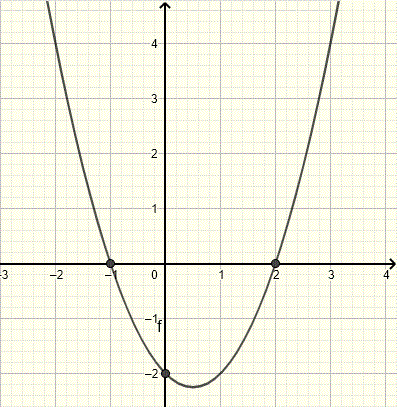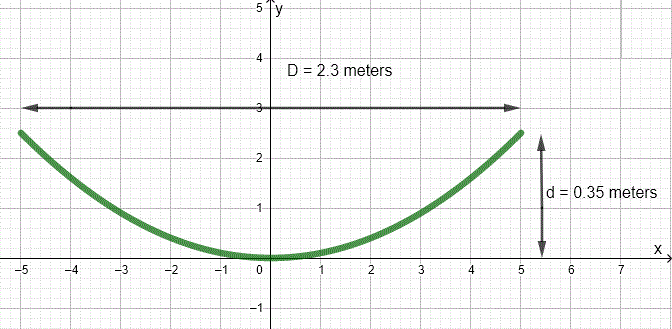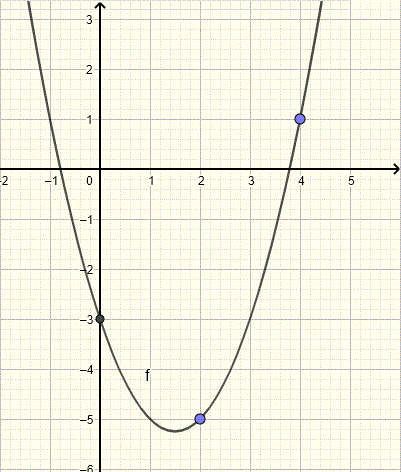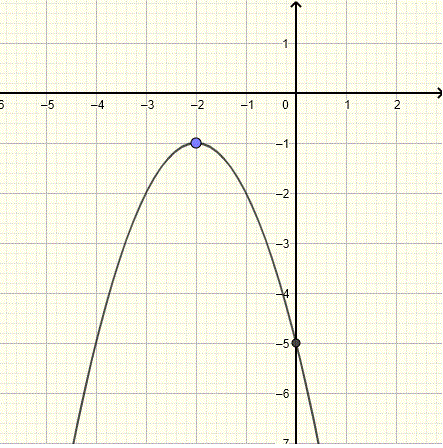# Find Equation of a Parabola from a Graph

Several methods are used to find equations of parabolas given their graphs. Examples are presented along with their detailed solutions and exercises.

## Examples with Detailed Solutions

Example 1 Graph of parabola given x and y intercepts
Find the equation of the parabola whose graph is shown below.Solution to Example 1
The graph has two x intercepts at $x = - 1$ and $x = 2$. Hence the equation of the parabola may be written as
$y = a(x + 1)(x - 2)$
We now need to find the coefficient $a$ using the y intercept at $(0,-2)$
$-2 = a(0 + 1)(0 - 2)$
Solve the above equation for $a$ to obtain
$a = 1$
The equation of the parabola whose graph is given above is
$y = (x + 1)(x - 2) = x^2 - x - 2$

Example 2 Graph of parabola given vertex and a point
Find the equation of the parabola whose graph is shown below.Solution to Example 2
The graph has a vertex at $(2,3)$. Hence the equation of the parabola in vertex form may be written as
$y = a(x - 2)^2 + 3$
We now use the y intercept at $(0,- 1)$ to find coefficient $a$.
$- 1 = a(0 - 2) + 3$
Solve the above for $a$ to obtain
$a = 2$
The equation of the parabola whose graph is shown above is
$y = 2(x - 2)^2 + 3$

Example 3 Graph of parabola given three points
Find the equation of the parabola whose graph is shown below.Solution to Example 3
The equation of a parabola with vertical axis may be written as
$y = a x^2 + b x + c$
Three points on the given graph of the parabola have coordinates $(-1,3), (0,-2)$ and $(2,6)$. Use these points to write the system of equations
$\begin{array}{lcl} a (-1)^2 + b (-1) + c & = & 3 \\ a (0)^2 + b (0) + c & = & -2 \\ a (2)^2 + b (2) + c & = & 6 \end{array}$
Simplify and rewrite as
$\begin{array}{lcl} a - b + c & = & 3 \\ c & = & -2 \\ 4 a + 2 b + c & = & 6 \end{array}$
Solve the above 3 by 3 system of linear equations to obtain the solution
$a = 3 , b=-2$ and $c=-2$
The equation of the parabola is given by
$y = 3 x^2 - 2 x - 2$

Example 4 Graph of parabola given diameter and depth
Find the equation of the parabolic reflector with diameter D = 2.3 meters and depth d = 0.35 meters and the coordinates of its focus.Solution to Example 4
The parabolic reflector has a vertex at the origin $(0,0)$, hence its equation is given by
$y = \dfrac{1}{4p} x^2$
The diameter and depth given may be interpreted as a point of coordinates $(D/2 , d) = (1.15 , 0.35)$ on the graph of the parabolic reflector. Hence the equation
$0.35 = \dfrac{1}{4p} (1.15)^2$
Solve the above equation for $p$ to find
$p = 0.94$
The equation of the parabola is given by
$y = 0.26 x^2$
The focus of the parabolic reflector is at the point
$(p , 0) = (0.94 , 0 )$

Find the equation of the parabola in each of the graphs below

1.2.3.1. $y = x^2-3x-3$
2. $y = - (x + 2)^2 - 1 = - x^2 -4x -5$
3. $y = (x-2)(x+6) = x^2 + 4x - 12$

## More References and Links to Parabola

Equation of a parabola.
Three Points Parabola Calculator.
Tutorial on
How Parabolic Dish Antennas work?
Tutorial on how to
Find The Focus of Parabolic Dish Antennas.
Use of parabolic shapes as
Parabolic Reflectors and Antannas.